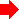Chapter 10: SIMULATION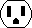## 10-6: The Gate Entity (ALS)The gate entity is the primary method of specifying behavior. It uses a truth-table to define the operational characteristics of a logic gate. Many behavioral descriptions need contain only a gate entity to be complete.

The gate entity is headed by the gate declaration statement and is followed by a body of information. The gate declaration contains a name and a list of exported simulation nodes (which are referenced in a higher level model description). The format of this statement is shown below:

 Format: gate name(signal1, signal2, signal3, ... signalN) Example: gate nor2(in1, in2, out) gate and3(a, b, c, output)

There is no limit on the number of signal names that can be placed in the list. If there is not enough room on a single line to accommodate all the names, simply continue the list on the next line.

### The i: and o: Statements (Input and Output)

The i: and o: statements are used to construct a logical truth table for a gate primitive. The signal names and logical assertions which follow the i: statement represent one of many possible input conditions. If the logic states of all the input signals match the conditions specified in the i: statement, the simulator will schedule the outputs for updating (as specified in the corresponding o: statement). The logical truth table for a two input AND gate is shown below:

```
gate and2(in1, in2, output)
i: in1=H in2=H  o: output=H
i: in1=L        o: output=L
i: in2=L        o: output=L
i:              o: output=X
```
The last line of the truth table represents a default condition in the event that none of the previous conditions are valid (e.g. in1=H and in2=X). It should be noted that the simulator examines the input conditions in the order that they appear in the truth table. If a valid input condition is found, the simulator schedules the corresponding output assignments and terminates the truth table search immediately.

### Signal References in the i: Statement

Besides testing the logical values of a signal, the i: statement can also compare them numerically. The format of a signal references, which follow the i: statement, is show below:

 Format: signal state_value or: signal other_signal Operators: = Test if equal ! Test if not equal < Test if less than > Test if greater than Example: node1 = H input1 ! input2 node3 < 16

There is no limit on the number of signal tests that can follow an i: statement. If there is not enough room on a single line to accommodate all the test conditions, the user can continue the list on the next line of the netlist.

### Signal References in the o: Statement

The signal references which follow the o: statement are used as registers for mathematical operations. It is possible to set a signal to a logic state and it is possible to perform mathematical operations on its contents. The format for signal references which follow the o: statement is shown below:

 Format: signal [ operand [ @ ] ] Operators: - decrement signal by value of operand = equate signal to value of operand + increment signal by value of operand - decrement signal by value of operand * multiply signal by value of operand / divide signal by value of operand % modulo signal by value of operand Strengths: 0 off 1 node 2 gate 3 VDD Example: qbar = H@3 out1 + 3 out + out1@4 node1 % modulus_node

It should be noted that the logic state of the operand can be directly specified (such as H, 3) or it can be indirectly addressed through a signal name (such as out1, modulus_node). In the indirect addressing case, the value of the signal specified as the operand is used in the mathematical calculations. The strength declaration is optional and if it is omitted, a default strength of 2 (gate) is assigned to the output signal.

### The t: Statement (Time Delay)

The propagation delay time (switching speed) of a gate can be set with the t: statement. The format of this statement is shown below:

 Format: t: = value { + = value ... } Mode: delta: fixed time delay in seconds linear: random time delay with uniform distribution random: probability function with values between 0 and 1.0 Example: t: delta=5.0e-9 t: delta=1.0e-9 + random=0.2

It is possible to combine multiple timing distributions by using the + operator between timing mode declarations. The timing values quoted in the statement should represent the situation where the gate is driving a single unit load (e.g. a minimum size inverter input).

The t: statement sets the timing parameters for each row in the truth table (i: and o: statement pair) that follows in the gate description. It is possible to set different rise and fall times for a gate by using more than one t: statement in the gate description. Assuming that a 2 input NAND gate had timing characteristics of t(lh) = 1.0 nanoseconds and t(hl) = 3.0 nanoseconds, the gate description for the device would be as follows:

```   gate nand2(in1, in2, output)
t: delta=3.0e-9
i: in1=H in2=H   o: output=L
t: delta=1.0e-9
i: in1=L         o: output=H
i: in2=L         o: output=H
```
This example shows that when both inputs are high, the output will go low after a delay of 3.0 nanoseconds and that if either input is low, the output will go high after a delay of 1.0 nanosecond.

### The Delta Timing Distribution of the t: Statement

The Delta timing distribution is used to specify a fixed, non-random delay. The format of a delta timing declaration is shown below:

 Format: delta = value Example: delta = 1.0 delta = 2.5e-9

The value associated with the delta declaration represents the fixed time delay in seconds (1.0 = 1 second, 2.5e-9 = 2.5 nanoseconds, etc.)

### The Linear Timing Distribution of the t: Statement

The Linear timing distribution is used to specify a random delay period that has a uniform probability distribution. The format of a linear timing declaration is shown below:

 Format: linear = value Example: linear = 1.0 linear = 2.0e-9

The value associated with the linear declaration represents the average delay time (in seconds) for the uniform distribution. This means that there is an equally likely chance that the delay time will lie anywhere between the bounds of 0 and 2 times the value specified.

### The Random Probability Function of the t: Statement

The random probability function enables the user to model things which occur on a percentage basis (e.g. bit error rate, packet routing). The format for random probability declaration is shown below:

 Format: random = value Example: random = 0.75 random = 0.25

The value associated with random declaration must be in the range 0.0 <= value <= 1.0. This value represents the percentage of the time that the event is intended to occur.

A gate which uses the random probability feature must be operated in parallel with another gate which has a common event driving input. Both these gates should have the same timing distributions associated with them. When the common input changes state, a probability trial is performed. If the probability value is less than or equal to the value specified in the random declaration, the gate containing the random declaration will have its priority temporarily upgraded and its outputs will change state before the outputs of the other gate. This feature gives the user some level of control (on a percentage basis) over which gate will process the input data first.

As an example, a system which models a communication channel that corrupts 1% of the data bytes that pass through it is shown below: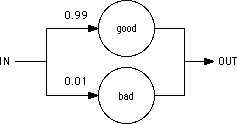``` model main(in, out) trans1: good(in, out) trans2: bad(in, out) gate good(in, out) t: delta=1.0e-6 i: in>0x00 o: out=in in=0x00 gate bad(in, out) t: delta=1.0e-6 + random=0.01 i: in>0x00 o: out=0xFF in=0x00 ```

The netlist describes a system where ASCII characters are represented by 0x01-0x7F. The value 0x00 indicates there is no data in the channel and the value 0xFF indicates a corrupted character. It is assumed that there is an external data source which supplies characters to the channel input. It should be noted that the random declaration is placed on only one of the two gate descriptions rather than both of them. Unpredictable events occur if the random declaration is placed on both gate descriptions.

### The Fanout Statement

The fanout statement is used to selectively enable/disable fanout calculations for a gate when the database is being compiled. The format for a fanout statement is shown below:

 Format: fanout = on or: fanout = off

When fanout calculation is enabled (the default setting for all gates), the simulator scans the database and determines the total load that the gate is driving. It then multiplies the gate timing parameters by an amount proportional to the load. If an inverter gate was found to have a propagation delay time of 1 nanosecond when driving a single inverter input, an instance of that gate would have a propagation delay time of 3 nanoseconds if it was driving a load equivalent to 3 inverter inputs.

If fanout calculation is turned off for a gate primitive, fanout calculations for all instances of that gate will be ignored. This feature allows the user to force switching times to a particular value and not have them modified by the simulator at run time.

The load statement is used to set the relative loading (capacitance) for an input or output signal. The format of a load statement is shown below:

 Format: load signal1 = value { signal2 = value ... } Example: load in1=2.0 in2=1.5 in3=1.95 load sa=2.5

The value associated with the signal represents the relative capacitance of the simulation node. When the timing parameters are specified for a gate description, it is assumed that they are chosen for the situation where the gate is driving a single (1.0) unit load such as a minimum size inverter input. The load command tells the simulator that some input structures are smaller or larger (more capacitive) than the reference standard. The simulator, by default, assumes that all signals associated with gate primitives have a load rating of 1.0 (unit load) unless they are overridden by a load statement.

### The Priority Statement

The priority statement is used to establish the scheduling priority for a gate primitive. The format for a priority statement is shown below:

 Format: priority = level Example: priority = 1 priority = 7

In the event that two gates are scheduled to update their outputs at exactly the same time, the gate with lowest priority level will be processed first. All gate primitives are assigned a default priority of 1 unless they contain random timing declarations in the gate description. In this case the primitive is assigned a default priority of 2. This base priority can be temporarily upgraded to a value of -1 if a random trial is successful during the course of a simulation run. The user is advised to leave the priority settings at their default values unless there is a specific requirement which demands priority readjustment.

### The Set Statement

The set statement is used to initialize signals to specific logic states before the simulation run takes place. The format for the set statement is shown below:

 Format: set signal1 = @ { } signal2 = @ { } Example: set input1=H@2 input2=L input3=X@0 set count=4 multiplier=5 divisor=7@2

If the user does not specify a strength value, the signal will be assigned a default logic strength of 3 (VDD). This default setting will override any gate output (because the default strength of 2 is used for gate outputs).

The user will find this feature useful in situations where some of the inputs to a logic gate need to be set to a fixed state for the entire duration of the simulation run. For example, the set and reset inputs of a flip flop should be tied low if these inputs are not being driven by any logic circuitry. All instances of a gate entity which contains a set statement will have their corresponding simulation nodes set to the desired state.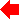Previous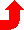Table of Contents Next